JAJSDO4C February   2017  – April 2020

PRODUCTION DATA.

1. 特長
2. アプリケーション
3. 概要
4. 改訂履歴
5. 概要（続き）
6. Device Comparison Table
7. Pin Configuration and Functions
8. Specifications
9. Detailed Description
1. 9.1 Overview
2. 9.2 Functional Block Diagrams
3. 9.3 Feature Description
4. 9.4 Device Functional Modes
5. 9.5 Register Maps
10. 10Application and Implementation
1. 10.1 Application Information
2. 10.2 Typical Application
11. 11Power Supply Recommendations
12. 12Layout
13. 13デバイスおよびドキュメントのサポート
14. 14メカニカル、パッケージ、および注文情報

• AAV|144

### 8.10 Typical Characteristics

Unless otherwise noted, all plots are at TA = 25°C, nominal supply voltages, fDAC = 8847.36MSPS, 12x interpolation, 0dBFS digital input, 40 mA full scale output current , LMFSHd = 84111 and PLL is disabled.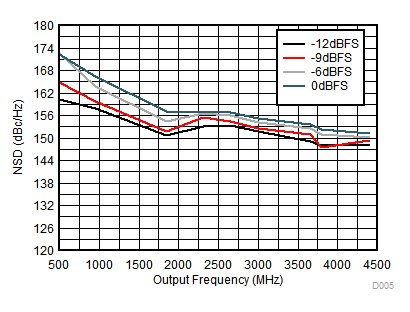Figure 1. NSD vs Output Frequency Over Input Scale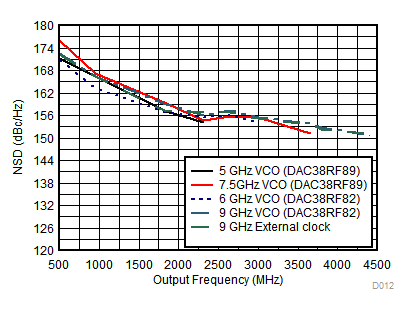Figure 3. NSD vs Output Frequency Over Clocking Option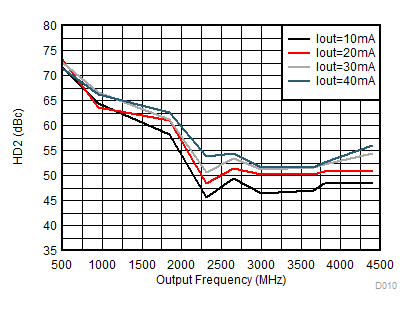Figure 5. HD2 vs Output Frequency Over Output Current IoutFS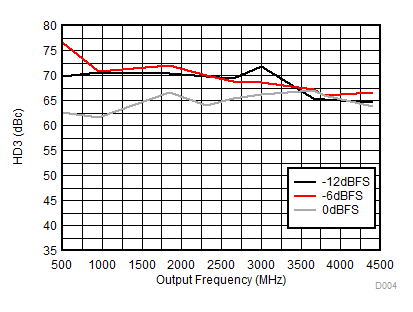Figure 7. HD3 vs Output Frequency Over Input Scale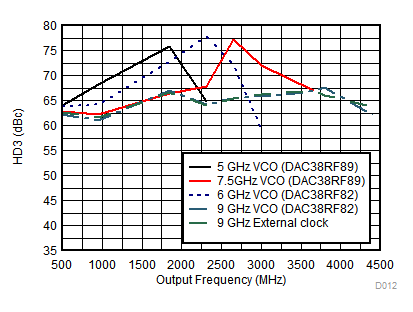Figure 9. HD3 vs Output Frequency Over Clocking Option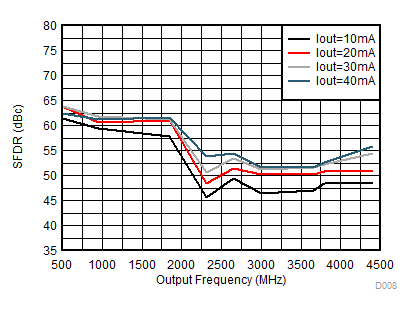Figure 11. SFDR vs Output Frequency Over Output Current IoutFS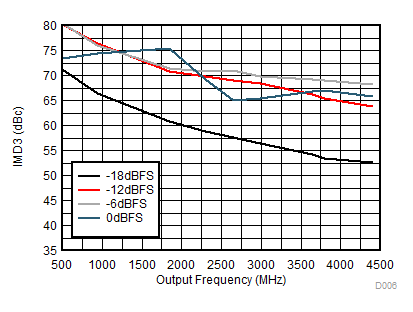Figure 13. IMD3 vs Output Frequency Over Input Scale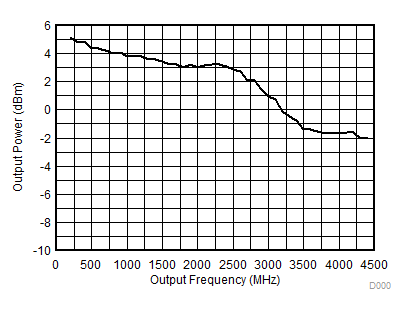Figure 15. Power vs Output Frequency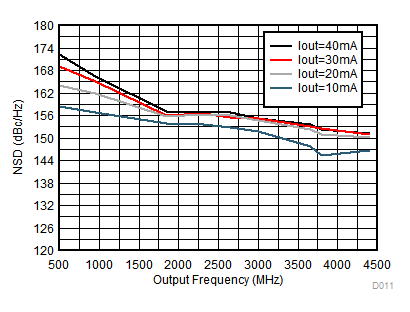Figure 2. NSD vs Output Frequency Over Output Current IoutFS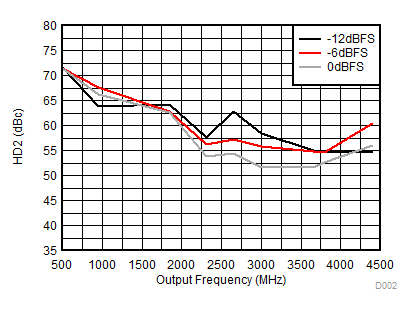Figure 4. HD2 vs Output Frequency Over Input Scale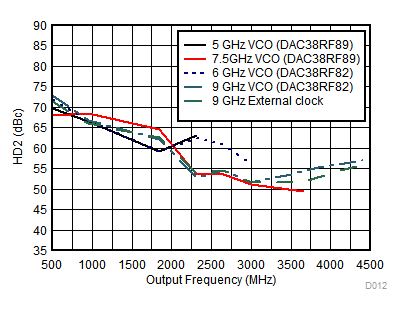Figure 6. HD2 vs Output Frequency Over Clocking Option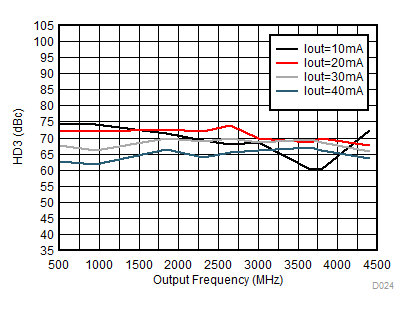Figure 8. HD3 vs Output Frequency Over Output Current IoutFS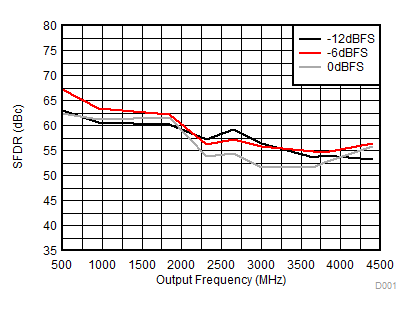Figure 10. SFDR vs Output Frequency Over Input Scale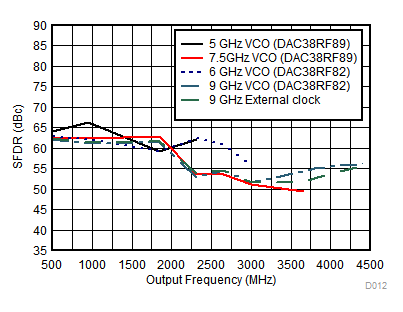Figure 12. SFDR vs Output Frequency Over Clocking Option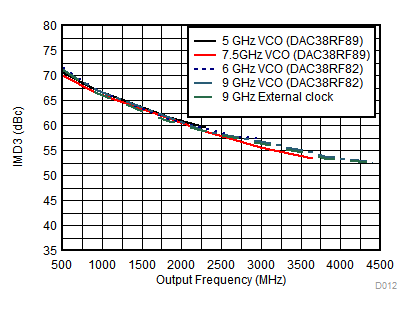-18 dBFS digital backoff
Figure 14. IMD3 vs Output Frequency Over Clocking Option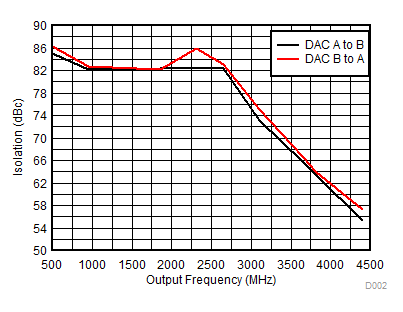Figure 16. Isolation vs Output Frequency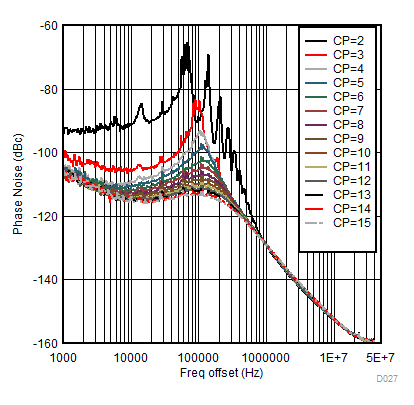VCO frequency = 8.85 GHz Measured at 1.8 GHz
Figure 17. DAC38RF82 VCO1 Phase Noise vs Offset Frequency Over Charge pump current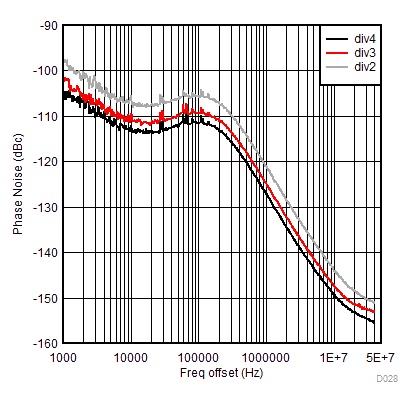VCO frequency = 8.85 GHz
Figure 19. DAC38RF82 VCO1 Output Clock Phase Noise vs Offset frequency Over Divider Ratio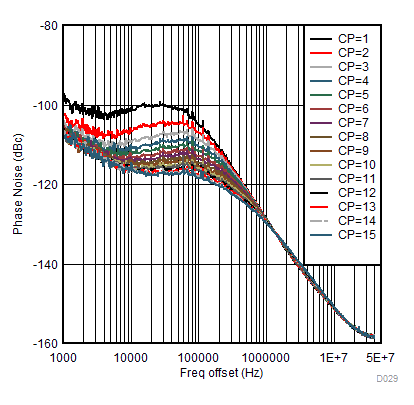VCO frequency = 5.9 GHz Measured at 1.8 GHz
Figure 21. DAC38RF82 VCO0 Phase Noise vs Offset Frequency Over Charge Pump Current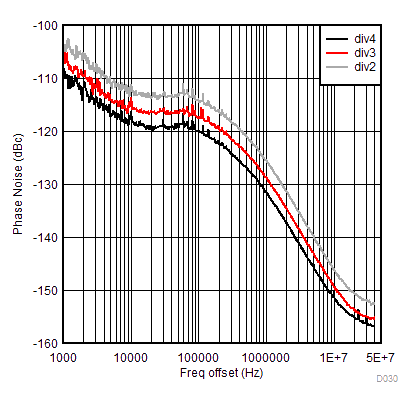VCO frequency = 5.9 GHz
Figure 23. DAC38RF82 VCO0 Output clock Phase Noise vs Offset Frequency Over Divider Ratio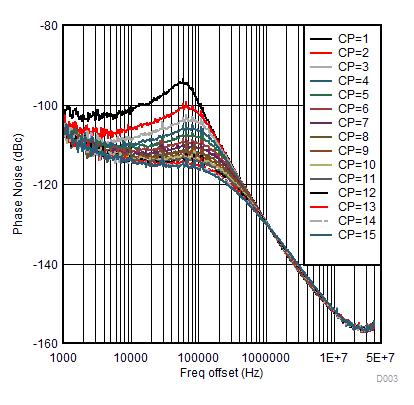VCO frequency = 7.5 GHz Measured at 1.8 GHz
Figure 18. DAC38RF89 VCO1 Phase Noise vs Offset Frequency Over Charge pump currentVCO frequency = 7.5 GHz
Figure 20. DAC38RF89 VCO1 Output Clock Phase Noise vs Offset frequency Over Divider Ratio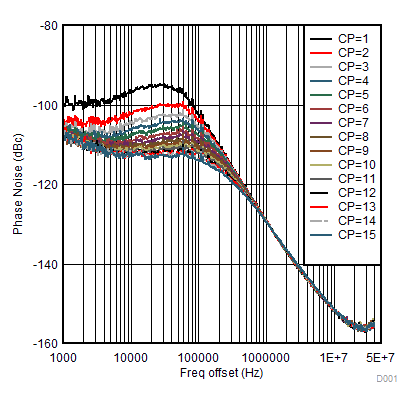VCO frequency = 5.0 GHz Measured at 1.8 GHz
Figure 22. DAC38RF89 VCO0 Phase Noise vs Offset Frequency Over Charge Pump Current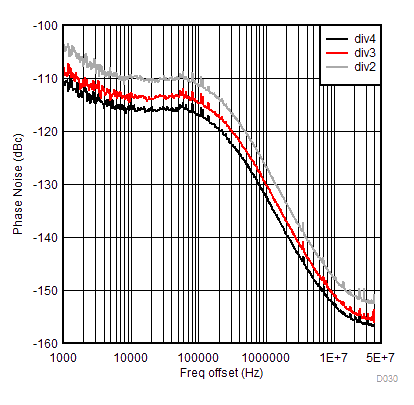VCO frequency = 5.0 GHz
Figure 24. DAC38RF89 VCO0 Output clock Phase Noise vs Offset Frequency Over Divider Ratio